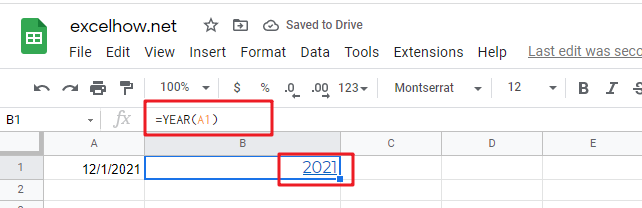# ExcelHow

This post will guide you how to use Google Sheets YEAR function with syntax and examples.

## Description

The Google Sheets YEAR function returns a four-digit year from a given date value, the year is returned as an integer ranging from 1900 to 9999. So you can use the YEAR function to get the year component of a date. You can also use this function to extract the year portion from an Excel date and feed the returned value into another formula.

The YEAR function can be used to get the year component of a data as a 4-digit number in google sheets. The purpose of this function is to get the yar from a data and the returned value is a year as 4-digit number.

The YEAR function is a build-in function in Google Sheets and it is categorized as a Date function.

## Syntax

The syntax of the YEAR function is as below:

=YEAR (serial_number)

Where the YEAR function arguments is:

• Serial_number -This is a required argument. A valid date value that contains the year component you want to extract. Dates should be entered by using the DATE function, or as results of other formulas or functions. For example, use DATE(2019,3,15) for the 15th day of March, 2019.

Note:

• A serial date is how the google sheets stores dates and it represents the number of days since 1900-01-01, so the January 1, 1900 date is serial number 1 by default.
• If the serial_number is not a valid date, it will return #VALUE! Error.

## Google Sheets YEAR Function Examples

The below examples will show you how to use google sheets YEAR Function to return an integer number that representing the year component of a given date.

1# get year of the date in Cell A1, enter the following formula in Cell B1.

`=YEAR(A1)`